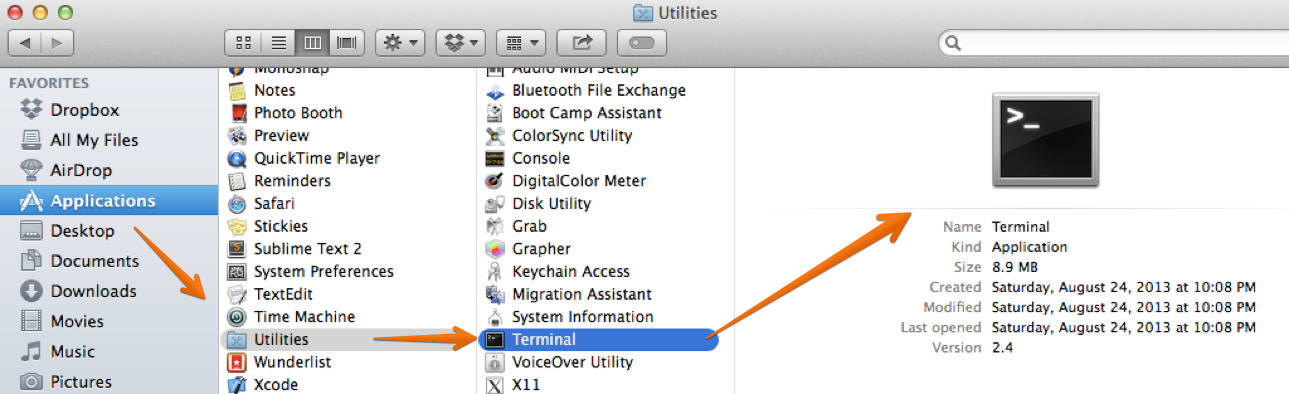# Numbers

Do you know basic math? Great, so does Ruby! Ruby uses the same adding, subtracting, multiplication and division that you do.

  2 + 2 => 4
9 - 3 => 6
2 * 3 => 6
4 / 2 => 2


Ruby can also perform logical operations, such as greater than > and less than <.

  4 > 2 => true
7 < 2 => false
3 >= 3 => true
0 <= 1 => true


You can try these simple commands yourself, but first you need to have Ruby installed on your computer.

Installing Ruby

If you’re using Mac OS X, Ruby is already installed. Hooray! Just open your Terminal application. To find terminal you can click the magnifying glass in the top right corner of your screen, then type Terminal and click the first result. (Or you can navigate to Applications - Utilities - Terminal and double click).If you’re using Linux, open up a shell, type irb and hit enter. And if you’re on Windows, open fxri from the Ruby section of your Start Menu.

As a third option, check out Repl.it (http://repl.it/languages/Ruby). This is a pretty fantastic tool that allows you to type code into your web browser. No installation required!

Next, type the letters irb into the terminal or shell screen and hit enter.

So, what is IRB anyway?

IRB stands for Interactive Ruby Shell. An Interactive Ruby Shell is like a little secret fort located inside your computer. You can use your IRB environment to play around with Ruby and learn different commands. Open up IRB (from a shell window or using www.repl.it/languages/Ruby and try typing some of these simple math calculations to see what the computer returns. Or try your own!

  2 + 2
4 < 7
5 > 10
7 / 4


Curious about the => arrows? Ruby engineers like to call these hash rockets. You will see that typing 3 + 2 or any other thing into IRB will always return a value, signified by the pointer =>.

A bit more math

Programming languages like Ruby can perform a lot of mathematical equations and expressions. Now that we can see how Ruby performs addition, subtraction, multiplication and division, we'll see how she handles exponents and the oh-so-cool modulo!

Exponents

Exponents tell a number how many times it should be multiplied. For example, 2 times 2 equals 4, 4 times 2 equals 8. So, 2^3 means 2 times 2 times 2, or 8. Don't worry about exponents if they are unfamiliar to you. They are not necessary to learn programming! Ruby uses two stars to signify an exponent math expression:

  2 ** 3 => 8
3 ** 2 => 9
10 ** 3 => 1000  #10 times 10 times 10!
# Also, the pound symbol is used for comments
# (and ignored by Ruby)


Modulo

In addition to these standard math operations, the computer has something called the modulo operator, which is represented using a percent symbol: %. The modulo’s job is to find the remainder after dividing one number with another. The remainder is what is left over, or what remains when you divide one big number by a smaller number. Let's look at an example.

9 divided by 3 would result in a modulo of 0, because there is no remainder. Since 3 divides evenly into 9 (three times) there are no numbers left over, and that is why 9 modulo 3 is 0. If we try it again with a different set of numbers, say 9 modulo 2, we would have a remainder (or a modulo operator) of 1. Because 9 divided by 2 equals 8, leaving a remainder of 1. Try these examples

  8 % 2 => 0
9 % 2 => 1
9 % 5 => 4


If exponents and modulos are too complex to understand right now, don't worry. Again, they are not a requirement for programming. It's important at this stage to simply understand that the computer can do simple math for you. Check out some examples below.